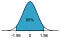1601161200

# ML: Hypothesis Testing

As data scientists, we need to know the proper way to build a hypothesis and test it with the tools that we learn. This post will guide you to build a proper and solid hypothesis.

## Minimum Description Length (MDL)

This is a simple concept. It means if you want to build a precise model, then the model will have small errors but it will have also the complexity of the model. If you want to build a simple model, then the model will have high errors. Always, the model complexity and the precision of the model is a trade-off because more bits are needed to build the precise model and more bits mean the complex model. Our goal is to build a model that has small errors and not a big complex model. This is related to Occam’s razor.

## Building Hypothesis and Confidence Interval

Let’s think about the example case, we are trying to measure the height of the students in the two different high schools and we know the result, means are 175cm for school A and 177cm for school B. This result is from the 50 students as a sample from each school. Can you tell the students in school B is taller than the students in school A? No. The answer is we don’t know. How does the data scientist answer those kinds of questions properly? Now, I will explain how we answer it step by step.#bioinformatics #hypothesis-testing #machine-learning #confidence-interval #data-science